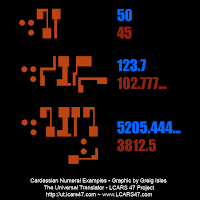The Universal Translator Blog is a sub-project of the LCARS 47 Development Project, a unique freeware canon accurate LCARS application suite.

## Friday, September 21, 2012

### Cardassian Alphabet - Part 3 (the numbers)

Here is my final post (for now), about Cardassian; as promised, I said I would discuss numbers, well here they are:

Cardassian uses ten of the letters of their alphabet for numeral glyphs, however they are usually flipped in relation to the rest of a text. Where it is necessary to distinguish numbers from letters, three dots in a triangular formation are used as a numeral determinative to mark the following glyphs as numbers.

## Function

In terms how the numerals write numbers, the Cardassians use a place value system similar to our own numerals; higher valued numbers come first with lower valued ones coming last. The only real difference is that the Cardassians use a base nine or nonary numeral system as standard: place values thus represent multiples of nine and not ten, as in a decimal system, so: 10 = 9, 20 = 18, 30 = 27 etc. 100 = 81, 1,000 = 729 and so forth. Cardassian numerals do also contain a glyph for 9, which can be used in decimal notation. However, this tends only to be used in mathematics and science; the Cardassians preferring base nine for everyday use.
Numbers are typically read and written in the same direction as the rest of a line of text; the standard punctuations marks of small circle, large circle and paragraph circle (refer to part 2) being used to indicate direction of reading.

## FractionsFractions, be they nonary or decimal, are written by using the same numeral glyphs as whole numbers, written underneath an whole number, at a 90º clockwise angle. Two dots may be written before a sequence of fractional numbers to indicate they are recurring. Where fractions occur alone without a leading whole number, they are written below a zero.

Well that about concludes all there is to say about Cardassian and their numerals.

For the Union!